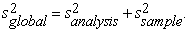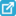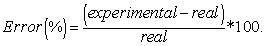﻿ An Exercise in Sampling: The Effect of Sample Size and Number of Samples on Sampling Error

### An Exercise in Sampling: The Effect of Sample Size and Number of Samples on Sampling Error

Juan M. Sanchez

World Journal of Chemical Education

## An Exercise in Sampling: The Effect of Sample Size and Number of Samples on Sampling Error

Juan M. SanchezDepartment of Chemistry, University of Girona, 17003-Girona, Spain

### Abstract

A simple and fast exercise is outlined to show science students the importance of determining the size of a sample and also the quantity of sample in order to minimize sampling error and improve accuracy, and to achieve a desired error.

• Juan M. Sanchez. An Exercise in Sampling: The Effect of Sample Size and Number of Samples on Sampling Error. World Journal of Chemical Education. Vol. 4, No. 2, 2016, pp 45-48. http://pubs.sciepub.com/wjce/4/2/3
• Sanchez, Juan M.. "An Exercise in Sampling: The Effect of Sample Size and Number of Samples on Sampling Error." World Journal of Chemical Education 4.2 (2016): 45-48.
• Sanchez, J. M. (2016). An Exercise in Sampling: The Effect of Sample Size and Number of Samples on Sampling Error. World Journal of Chemical Education, 4(2), 45-48.
• Sanchez, Juan M.. "An Exercise in Sampling: The Effect of Sample Size and Number of Samples on Sampling Error." World Journal of Chemical Education 4, no. 2 (2016): 45-48.

 Import into BibTeX Import into EndNote Import into RefMan Import into RefWorks

1
Prev Next

### 1. Introduction

Progress in instrumental analysis in the last few decades has resulted in an increase in the quality of analytical results mainly in terms of data precision and the lowering of detection limits. The subsequent data treatment has also been improved by the use of chemometric techniques. However, it is not unusual to obtain widely differing results when the laboratories participate in the sampling stage of an inter-laboratory study.

Sampling is a critically important stage in any analytical procedure that aims to reduce the amount of material to be analyzed to a representative portion that can be manipulated in the laboratory. This stage determines the overall analytical result. If we only take into account random errors in data variability, two main contributions to the global error come into play: one is from the sample and the other is from the analysis,(1)

The error in the analysis is usually between 0.1-1% whereas the sample error can be higher than 100% for samples with considerable heterogeneity.

Despite its importance in the global error of the analytical procedure, sampling receives less attention in the analytical curriculum than its importance warrants. Current introductory analytical texts do address the importance of sampling [1, 2, 3] but fail to give the detailed treatment of Laitinen and Harris’ classic text  and they make little mention of the problems encountered in obtaining representative samples.

Another problem is the difficult in effectively communicating the importance of sampling to students in a standard introductory analytical course. It is still common practice to provide students with a prepared sample in the laboratory, which lessens student awareness as to the importance of sampling. To address this problem, laboratory and classroom exercises should be developed to help students understand and appreciate the challenge involved in obtaining an analytical sample that is representative of the material.

In this paper a simple classroom exercise is designed for analytical courses which introduces the basic concepts of sampling and statistical data analysis. This exercise is a variation of the experiment and lecture demonstration developed by Kratochvil et al.  and shows the effect of sample size and the number of samples on sampling error and visualize in a simple way how the variation of these two parameters impact on the sampling error. There are many other classroom exercises that also cover the basics of statistical analysis of a data set and show the error introduced by sampling [6-13], but they usually are not focus to show the effect of the quantity of samples and their size in the sampling error obtained, and the importance of these two parametrers in chemical analysis. The exercise outlined here has been used in our Chemical Analysis course at the University of Girona for the past ten years. The data collection and calculation steps can easily be performed in a 45-minute class. The response from students suggests that it is of considerable use in helping them to understand the importance of correct sampling.

### 2. Sample Exercise

The exercise is quite simple and only requires a bag of fruit-flavored candies (about 2 Kg for a classroom of 27 students in 9 groups of 3 people), which can later be given to the students as a reward, and non-reusable plastic beakers of three different sizes.

In our classes we use candies in the shape of a magnetic stirrer bar (about 1.5 cm length and 0.5 cm diameter) with 6 different colors. The exact color composition of the mixture of candies is previously determined but the result is not made available to the students until they have finished all their calculations.

As one of the objectives is to assess the effect of sample size, three different sizes of non-reusable beakers are used. The smaller beaker has a capacity for around 20 candies, the medium beaker for around 40, and the largest beaker allows around 60 pieces of candy.

Each group of students receives one beaker of each size and scoops up three samples from the candy bag by filling all of their beakers to the top. The number of candies per color, the total number, and the percentages is calculated for each sample. Q-testing is performed to analyze apparent outliers. An example of class results for this exercise is shown in Table 1.

#### Table 1. Example of class results with color percentages for each sample size. Real composition of the sample: white 48,0 %, yellow 12,2 %, blue 18,9 %, red 4,1%, green 9,0 %, and orange 7,8 %. Sample sizes were as noted in Figure 1Download asPowerPoint Slide

Veiw figureView current table in a new windowDownload asVeiw figureFigures index
Figure 1. Box-plot comparison to evaluate the data distribution for small (a), medium (b) and large (c) sample sizes. Results obtained from a class of 9 groups; sizes of the samples were 19±3 (small), 43±3 (medium), and 58±4 (large) candies

### 3. Evaluation of the Data

3.1. Sample Size

First of all, each group of students is asked to use their own data to assess whether there is an effect of the sample size on the results obtained. The first impression students obtain from their results is that each size of beaker gives different percentages for each color (Table 1). This allows them to realize that sample size has a significant effect on the error of the data calculated.

Some of the years that I have used this exercise students were taught sampling theory beforehand and this helped them to conclude that the results for the higher sample size must give a greater level of precision. Other years, students were asked to do the exercise before learning the theory and I have observed that they randomly selected one or other of the sizes after comparing their results with neighbor groups. These results ascertain the importance of having a good theoretical background in making correct decisions about the interpretation of laboratory data.

In a second step, students are asked to report their results using a spreadsheet, which is provided to all students. From this data they have to calculate the average percentage, standard deviation and relative standard deviation (RSD) for all samples taken with the same beaker size from the different groups (Table 1). Using standard deviation to test for significant differences, students easily see that as the sample size increases, the standard deviation in the data decreases (i.e. better precision). A box-plot graph of the data obtained for a whole class (Figure 1) helps to illustrate this.

3.2. Number of Samples

The results of the whole class are also used to assess the effect of the number of samples on the accuracy of results. The real color composition in the bag is delivered to the students. They are asked to determine the accuracy of their group data by calculating the error in their predictions as a percentage for the three sample sizes:(2)Download asVeiw figureFigures index
Figure 2. Evolution of the percentage of error in the measurement of the color composition in large sample size as the number of samples used in the measurement increases

Once this has been done, they compare the results with the errors obtained when the average of the mean results of each group are calculated (Table 1, 9 samples for each size). The results obtained show that the errors are always smaller when the mean of all samples are included than just one single sample. Furthermore, as can be seen in Figure 2, we observe that the error becomes smaller as a larger number of samples is introduced into the calculations. Error calculation in Figure 2 was determined by taking the average of n randomly selected groups from the total group.

### 4. Conclusions

The use of a simple class exercise using a mixture of candies of the same shape and size, but each with a different color and composition, is an effective way to show science students the significant effect that two basic aspects of sampling -sample size and the number of replicates analyzed- have on the accuracy and precision achieved in laboratory measurements.

First, a comparison of the results obtained using three different sample sizes (with only one replicate for each one) shows students how very different results are often obtained from the same sample. This preliminary evaluation does not require any calculation other than the determination of percentages of each color in the mixture. It is, however, a very simple way of showing students that a correct choice of the sample size is a determining factor in achieving good results.

Second, the effect of the number of replicates can be simply evaluated by choosing a random combination of the results obtained from different groups. In this situation a simple calculation based on equation 2 is required to assess the percentage of error obtained for different numbers of replicates. A significant reduction in the error in the measurement is always obtained when each group compare their single results with any combination of more than one replicate, demonstrating the need to use replicates to improve the accuracy of the results and reduce the error of the measurements. When different numbers of replicates are evaluated, a decrease in the measurement error is obtained. It also helps to show students that the increase in the number of replicates reduces the error but that this decrease is not linear and the improvement in the error is reduced as the number of replicates increases.

The exercise developed here represents a simple and easy way of showing students the importance of correct sampling in any analytical procedure. This exercise gives better results in teaching students the effect of sample size and number on the error of a measurement than any theoretical explanation. I believe that this kind of simple exercise has educational value as they introduce students to complicated mathematical and statistical concepts through the use of simple calculations.

### References

  Harris, D.C., Quantitative Chemical Analysis, W.H. Freeman and Co., New York, 2007.In article  Skoog, D.A., West, D.M., Holler, F.J., Crouch, S.R., Fundamentals of Analytical Chemistry, 8th ed., Brooks/Cole, Belmont, CA, 2014.In article  Christian, G.D., Analytical Chemistry, Wiley, 2004.In article  Laitinen, H.A. and Harris, W.E., Chemical Analysis, McGraw-Hill, New York, 1975.In article  Kratochvil, B., Reid, R.S., Harris, W.E., “Sampling error in a particulate mixture: an analytical chemistry experiment”, Journal of Chemical Education, 57(7), 518-520, 1980.In article View Article  Bauer C.F., “Sampling error lecture demonstration”, Journal of Chemical Education, 62(3), 253, 1985.In article View Article  Stone, C.A. and Mumaw, L.D., “Practical experiments is statistics”, Journal of Chemical Education, 72(6), 518-524, 1995.In article View Article  Salzsieder, J.C., “Statistical analysis experiment for the freshman chemistry lab”, Journal of Chemical Education, 72(7), 623, 1995.In article View Article  Vitt, J.E. and Engstrom, R.C., “Effect of sample size on sampling error: an experiment for introductory analytical chemistry”, Journal of Chemical Education, 76(1), 99-100, 1999.In article View Article  Ross, M.R., Bacon, D.W., Wolsey, W.C., “A classroom exercise in sampling technique”, Journal of Chemical Education, 77(8), 1015-1016, 2000.In article View Article  Hartman, J.R.,” An in-class experiment to illustrate the importance of sampling techniques and statistical analysis of data to quantitative analysis students”, Journal of Chemical Education, 77(8), 1017-1018, 2000.In article View Article  Canaes, L.S., Brancalion, M.L., Rossi, A.V., Rath, S., “Using candy samples to learn about sampling techniques and statistical data evaluation”, Journal of Chemical Education, 85(8), 1083, 2008.In article View Article  Logue, B.A. and Youso, S.L., “A laboratory exercise to demonstrate the theory and practice of analytical sampling”, Journal of Chemical Education, 87(3), 316-319, 2010.In article View Article